# 特殊人群疏散特性的元胞自动机模拟Cellular Automata Simulation of Evacuation Characteristics of Special Pedestrian

• 全文下载: PDF(1408KB)    PP.24-31   DOI: 10.12677/MOS.2018.71004
• 下载量: 613  浏览量: 1,527   国家自然科学基金支持

Based on cellular automata theory, the cellular automata model describing the normal pedestrian evacuation is extended to study the evacuation scenario including special pedestrians. Through analyzing the psychological characteristics of special pedestrians (This article mainly refers to the pedestrian who are unable to move freely), a cellular automaton model is established. The numerical simulations are performed to investigate the influence of the proportion and location distribution of special pedestrian on the evacuation process in the hall. The spatio-temporal dynamic characteristic of pedestrian evacuation process is also discussed. The results show that the effect of the special crowd on the whole evacuation efficiency is related to the total pedestrian density. At low density, this effect on the evacuation efficiency is not obvious, but with the increase of density, the proportion of special pedestrians will lead to a significant decrease in the evacuation efficiency. In addition, the location distribution of the special pedestrians will also affect the evacuation efficiency. Especially at high density, compared with the random distribution of the special pedestrians, to put them in a certain position and separate them from the normal pedestrians will reduce the overall evacuation time remarkably.

1. 引言

2. 模型

${p}_{i,j}=0\text{\hspace{0.17em}}\left(x,y\right)\notin {{S}^{\prime }}_{i,j}$ ；(行人下一步移动位置不为空，则停在原位置)

${p}_{i,j}=1/|{{S}^{\prime }}_{i,j}|\left(x,y\right)\in {{S}^{\prime }}_{i,j}$ ；(行人下一步移动位置为空，则等概率选择方向移动)

${p}_{i,j}=1\left(i,j\right)=\left({i}_{0},{j}_{0}\right)$ 。(行人在出口位置，则下一步一定移出系统)， $|{{S}^{\prime }}_{i,j}|$ 表示 ${{S}^{\prime }}_{i,j}$ 中元素个数。

3. 模拟结果与讨论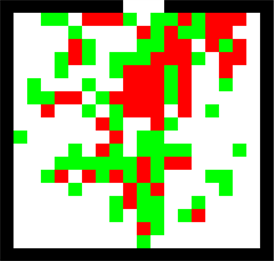Figure 1. Sketch of model(a)(b)

Figure 2. The selectable movement directions. (a) Normal pedestrians; (b) Pedestrians who are unable to move freely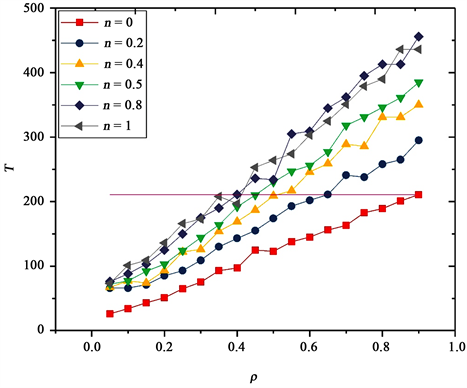Figure 3. Relationship between evacuation time T and density ρ under n = 0, 0.2, 0.4, 0.5, 0.8, 1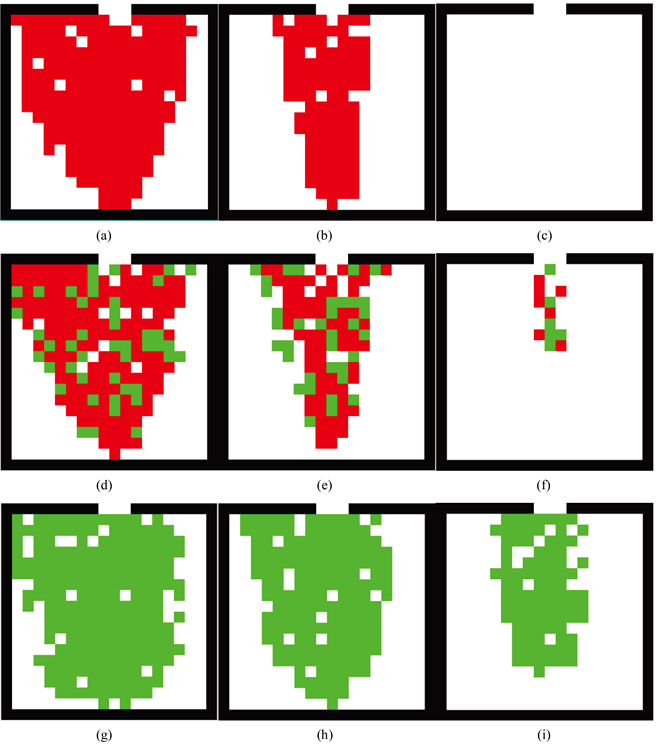Figure 4. The typical spatial-temporal patterns as ρ = 0.7. n = 0: (a) T = 40 s, (b) T = 100 s, (c) T = 190 s; n = 0.2: (d) T = 40 s, (e) T = 100 s, (f) T = 190 s; n = 1: (g) T = 40 s, (h) T = 100 s, (i) T = 190 s(a)(b)

Figure 5. The typical spatial-temporal patterns as ρ = 0.7. (a) T = 10 s; (b) T = 40 s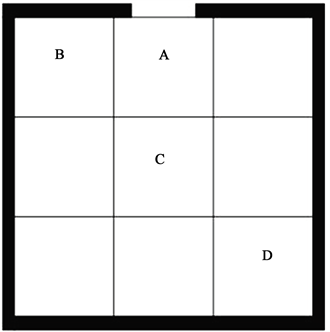Figure 6. The distribution of pedestrians who are unable to move freely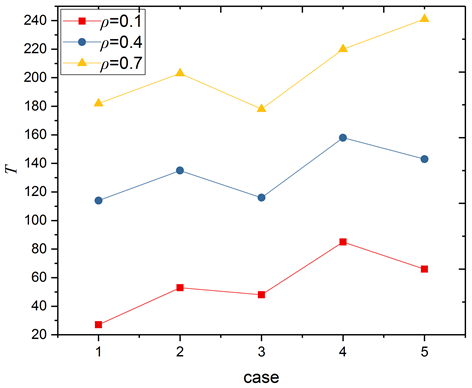Figure 7. Relationship between evacuation time T and case under n = 0.2, ρ = 0.1, 0.4, 0.7

4. 结论

1) 人群中加入行动不便行人将会影响行人总体的疏散时间。

2) 低密度下，行人不便行人对人群疏散效率影响不明显，随着密度的增加，行动不便行人所占比例的增大则会导致混合人群的疏散效率显著下降。

3) 高密度下，将行动不便行人和正常行人分开，会大幅提高疏散效率，且将行动不便行人置于出口附近或房间中央位置，对人群疏散最有利。

  Helbing, D. (2000) Traffic and Related Self-Driven Many-Particle Systems. Physics, 73, 1067-1141.  Henderson, L.F. (1971) The Statistics of Crowd Fluids. Nature, 229, 381-383. https://doi.org/10.1038/229381a0  Hoogendoorn, S.P. (2015) Continuum Theory for Pedestrian Traffic Flow: Local Mute Choice Modelling and Its Implications. Transportation Research Pact C, 59, 183-197. https://doi.org/10.1016/j.trc.2015.05.003  Helbing, D. and Molnár, P. (1995) Social Force Model for Pedestrian Dynamics. Physical Review E: Statistical Physics Plasmas Fluids & Related Interdisciplinary Topics, 51, 4282. https://doi.org/10.1103/PhysRevE.51.4282  Helbing, D., Farkas, I. and Vicsek, T. (2000) Simulating Dynamical Features of Escape Panic. Nature, 407, 487-490. https://doi.org/10.1038/35035023  Chraibi, M., Seyfried, A. and Schadschneider, A. (2010) Generalized Centrifugal-Force Model for Pedestrian Dynamics. Physical Review E: Statistical Nonlinear & Soft Matter Physics, 82, 046111. https://doi.org/10.1103/PhysRevE.82.046111  Burstedde, C., Klauck, K., Schadschneider, A., et al. (2001) Simulation of Pedestrian Dynamics Using a Two-Dimensional Cellular Automaton. Physica A: Statistical Mechanics & Its Applications, 295, 507-525. https://doi.org/10.1016/S0378-4371(01)00141-8  Kirchner, A. and Schadschneider, A. (2002) Simulation of Evacuation Processes Using a Bionics-Inspired Cellular Automaton Model for Pedestrian Dynamics. Physica A: Statistical Mechanics & Its Applications, 312, 260-276. https://doi.org/10.1016/S0378-4371(02)00857-9  Zhang, P., Jian, X.X., Wong, S.C., et al. (2012) Potential Field Cellular Automata Model for Pedestrian Flow. Physical Review E: Statistical Nonlinear & Soft Matter Physics, 85, 021119. https://doi.org/10.1103/PhysRevE.85.021119  Jian, X.X., Wong, S.C., Zhang, P., et al. (2014) Perceived Cost Potential Field Cellular Automata Model with an Aggregated Force Field for Pedestrian Dynamics. Transportation Research Part C: Emerging Technologies, 42, 200-210. https://doi.org/10.1016/j.trc.2014.01.018  Tajima, Y. and Nagatani, T. (2001) Scaling Behavior of Crowd Flow Outside a Hall. Physica A: Statistical Mechanics & Its Applications, 292, 545-554. https://doi.org/10.1016/S0378-4371(00)00630-0  Helbing, D., Isobe, M., Nagatani, T., et al. (2003) Lattice Gas Simulation of Experimentally Studied Evacuation Dynamics. Physical Review E, 67, 067101. https://doi.org/10.1103/PhysRevE.67.067101  Kuang, H., Li, X., Song, T., et al. (2008) Analysis of Pedestrian Dynamics in Counter Flow via an Extended Lattice Gas Model. Physical Review E: Statistical Nonlinear & Soft Matter Physics, 78, 066117. https://doi.org/10.1103/PhysRevE.78.066117  Blue, V.J. and Adler, J.L. (2001) Cellular Automata Microsimulation for Modeling Bi-Directional Pedestrian Walkways. Transportation Research Part B: Methodological, 35, 293-312. https://doi.org/10.1016/S0191-2615(99)00052-1  杨立中, 方伟峰, 黄锐, 等. 基于元胞自动机的火灾中人员逃生的模型[J]. 科学通报, 2002, 47(12): 896-901.  郑美容. 基于元胞自动机的人员疏散行为模拟研究[J]. 陕西理工学院学报(自科版), 2016, 32(2): 39-44.  林煌涛. 基于元胞自动机模型的礼堂人群疏散仿真[J]. 现代计算机, 2013(13): 41-44.  王浩. 基于多智能体的地铁车站行人疏散仿真及其内部设施布局优化[D]: [硕士学位论文]. 成都: 电子科技大学, 2015.  张艳芬. 大型客运站突发事件客流疏散仿真方法的研究[D]: [硕士学位论文]. 北京: 北京交通大学, 2013.  姜子港, 刘晓栋, 霍非舟, 等. 基于Pathfinder的商场人员疏散仿真[J]. 火灾科学, 2014, 23(3): 175-181.  田玉敏. 特殊人群疏散行为及疏散设计的研究[J]. 灾害学, 2013, 28(3): 91-94.  薛李生辉, 王坚, 王超, 等. 考虑个体差异的改进PSO人群疏散模型[J]. 中国公共安全: 学术版, 2016(3).  姜传胜, 郑双忠, 袁斐. 残疾人疏散安全研究现状与发展趋势[J]. 中国安全科学学报, 2009, 19(3): 161-166.